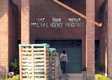### Syllabus for Written exam for Ph. D. and M. Tech. admissions 2017- 18There will be two sections in the written exam. One section is common to all which has mathematic questions (see the syllabus). The other section will have questions from different streams. Student belongs to particular steam will answer the corresponding stream questions. The questions can be from the basic relevant subjects with respect to their stream.

Syllabus for Mathematics

• Basic linear algebra (matrices & determinants)

• Basic integral and differential calculus

• Basic vector calculus

• Basic Probability and statistics

Sri Sivakumar
DPGC, Convenor
Materials Science Programme
IIT Kanpur